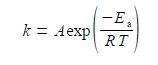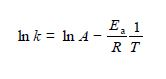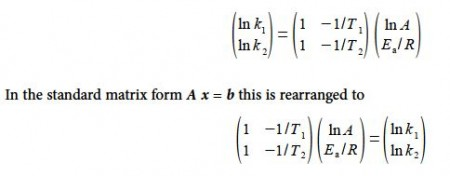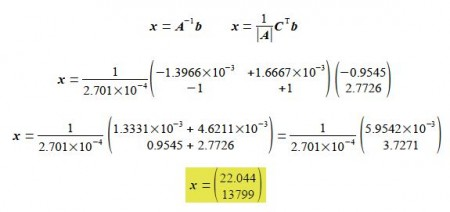# What is the Speed of Chemical Reactions?

The Arrhenius equation is an important equation in chemical reactions kineticswhere k is the rate constant, A is the pre-exponential factor, Ea the activation energy, T is the temperature in kelvin, and R is the gas constant. This can be rearranged into a linear form by taking natural logarithms of both sides,If the value of k can be measured at a series of temperatures T, then the two unknown parameters A and Ea. can be calculated by solving the resulting simultaneous equations.

The initial stage of the chemical reactions between ammonia and nitrous oxide follows second order kinetics. Given that the rate constant at 600 K is 0.385 L mol-1 s-1 and at 716 K is 16.0 L mol-1 s-1, calculate the activation energy Ea, and the pre-exponential factor A to 3 significant figures.

SolutionSubstituting in the experimental values without units for clarity, and for the moment working to extra significant figures to avoid rounding errors,Solving for the unknowns matrix x using,Thus In A = 22.044 and the pre-exponential factor to three significant figures is,

A = 175 x 109 L mol-1 s-1

Ea /R = 13,799 so Ea = 13,799 x 8.314 J mol-1 K-1 = 114.72 x 103 J mol-1 K-1 or in the conventional units and to three significant figures,

Ea = 115 kJ mol-1